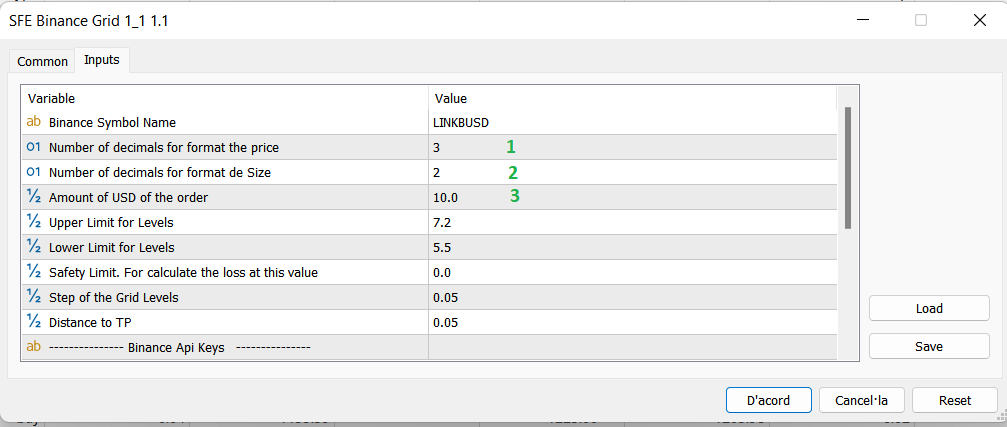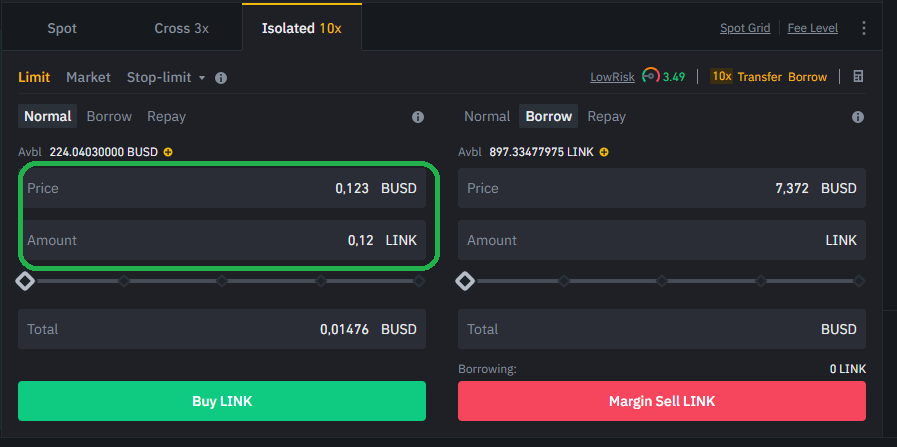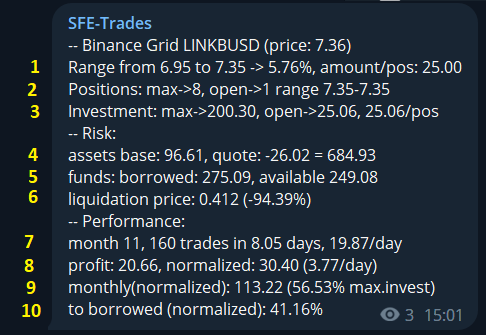visit previous post for general introduction.  https://www.mql5.com/en/blogs/post/751047

Product's page: https://www.mql5.com/en/market/product/89642

The parameters has changed a bit on version 1.1.The number of decimals of the price must be entered (1)

The number of decimals of the size / amount  must be entered (2)

Now, insted the size of the position, you enter the amount of USD to use (3).

(the values of (1) (2) can be cheched in your binance platform, in margin trading, and look how many decimals you can use for price or size)The current information you will receive each time a grid trade is closed is:Grid parameters:

1. the current range used in the grid and the amount assigned to each position.

2. how many positions can open this grid range and how many positions are opened right now.

3. which is the max. investment of the current grid range.

Risk:

4. which is the value of the current assets (crypto + \$\$).

5. borrowed \$\$ funds if any and available part of these funds.

6. liquidation price with the current situation (current trades, current borrowed)

Performance:

7. in the current month, how many trades since started

8. in the current month, profit. Normalized means using the current amount parameter on closed trades.

9. profit normalized projected to the whole month. and relation with the current max. investment.

10. relation with the current borrowed amount (if any)

Share it with friends: